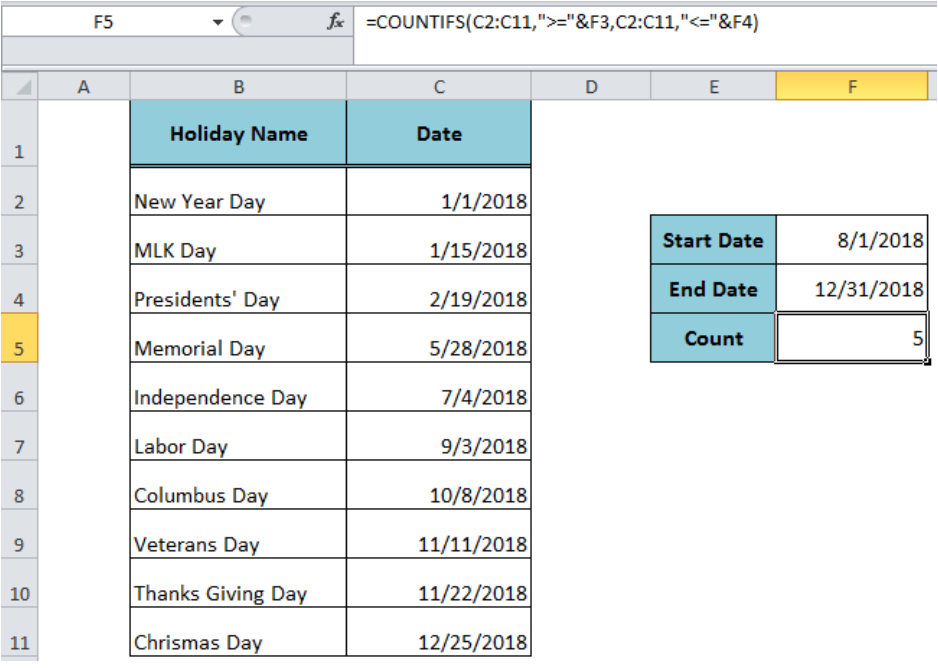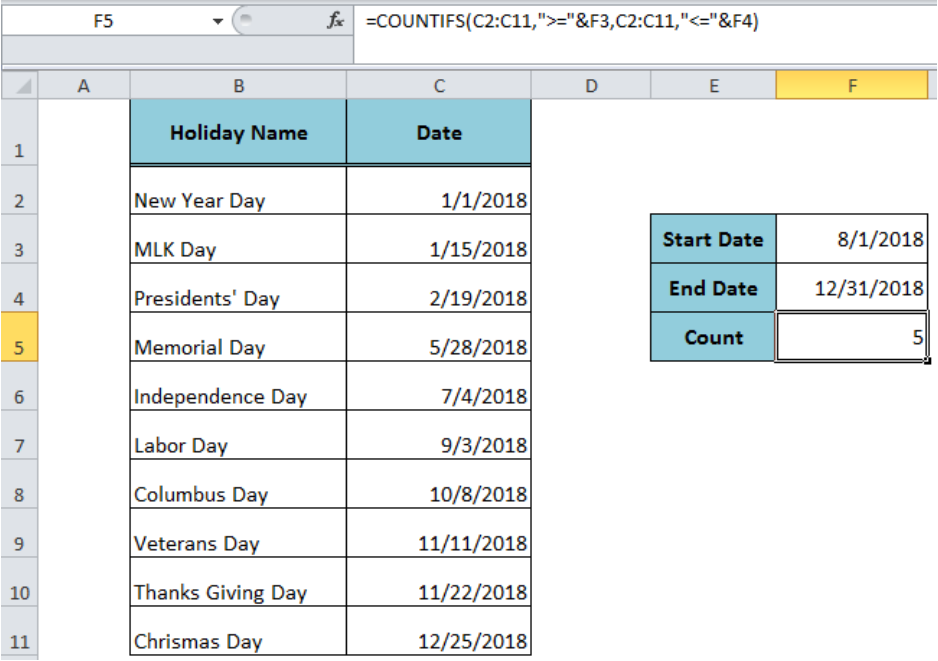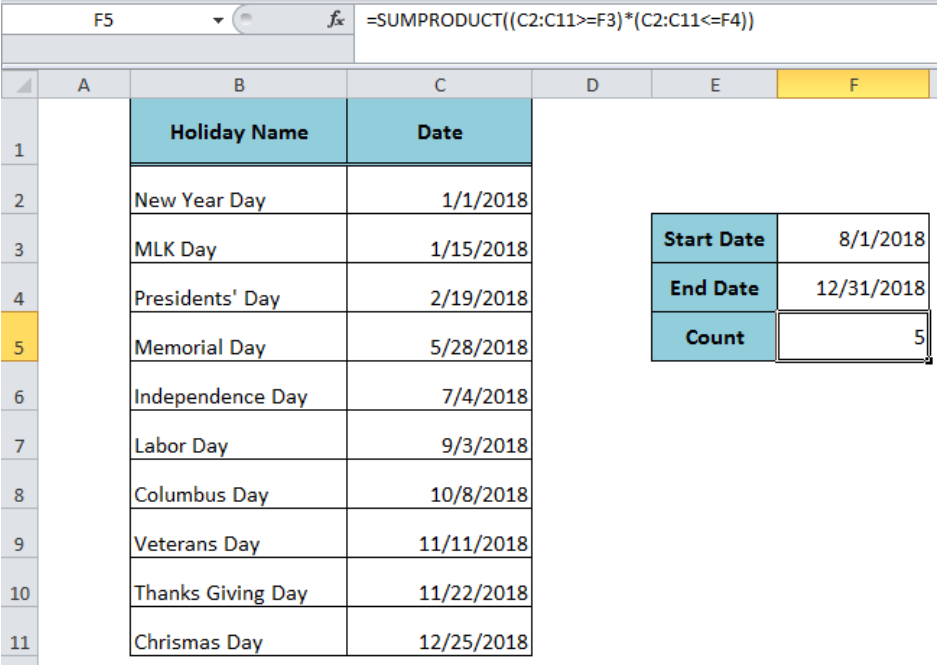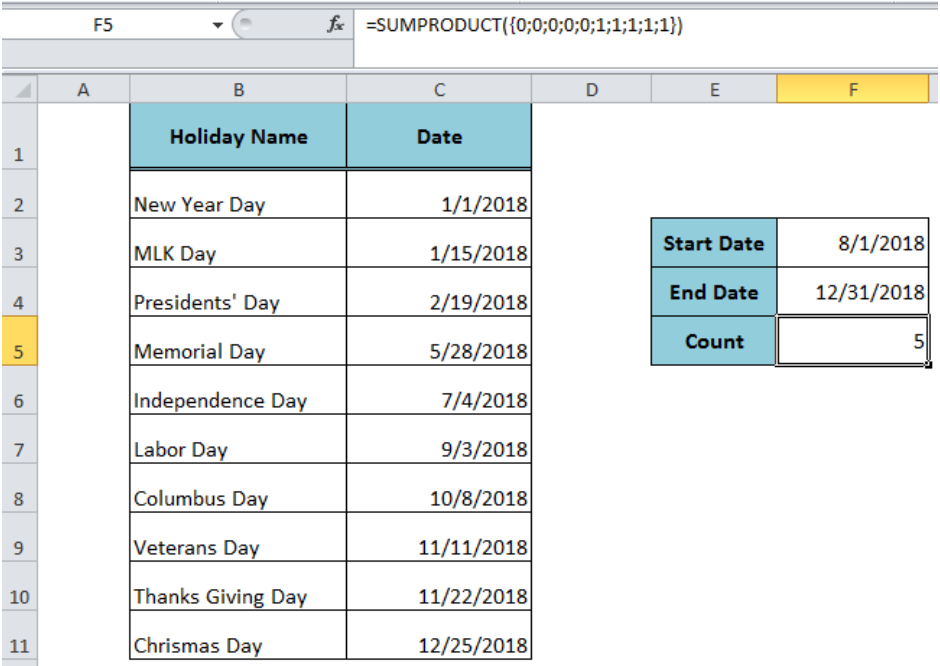Get instant live expert help with Excel or Google Sheets“My Excelchat expert helped me in less than 20 minutes, saving me what would have been 5 hours of work!”

#### Post your problem and you’ll get expert help in seconds.

Your message must be at least 40 characters
Our professional experts are available now. Your privacy is guaranteed.

# Count holidays between two dates

We often need to count holidays between two dates while developing a timeline for a project. In Excel, we can achieve this by using the COUNTIFS or the SUMPRODUCT function when we have a list of holidays’ dates in a range. This article will explain the process.Figure 1. Counting Holidays Between Two Dates

## Counting Holidays Using the COUNTIFS Function

When holidays’ dates are listed in a range then we can count holidays between two dates using the COUNTIFS function. The COUNTIFS function will count the cells in range C2: C11, where dates fall between the start date and end date criteria, given in cells F2 and F3 as per our example in the following formula in cell F5;

`=COUNTIFS(C2:C11,">="&F3,C2:C11,"<="&F4)`Figure 2. Counting Holidays Using the COUNTIFS Function

## Counting Holidays Using the SUMPRODUCT Function

Using the SUMPRODUCT function we can count the cells in range C2: C11 that meet the two expressions, greater than equal to start date `(>=start_date)` and less than equal to the end date `(<=end_date)`. The following formula will be used in cell F5 to count the holidays between two dates;

`=SUMPRODUCT((C2:C11>=F3)*(C2:C11<=F4))`Figure 3. Counting Holidays Between Dates Using the SUMPRODUCT Function

When we test each expression on a single array it generates an array of TRUE and FALSE logical values, such as;

Expression `(C2: C11>=F3)` returns an array of logical values as follows;

`{FALSE;FALSE;FALSE;FALSE;FALSE;TRUE;TRUE;TRUE;TRUE;TRUE}`

And expression `(C2: C11<=F4)` returns an array of logical values as follows;

`{TRUE;TRUE;TRUE;TRUE;TRUE;TRUE;TRUE;TRUE;TRUE;TRUE}`

As per boolean logic, when these arrays of TRUE and FALSE are multiplied they generate an array of 1s and 0s and the SUMPRODUCT sums the values inside it. To see the working of this formula in Excel formula bar, select both expressions inside the SUMPRODUCT function and press F9, such as;

`=SUMPRODUCT({0;0;0;0;0;1;1;1;1;1})`Figure 4. Working of the SUMPRODUCT Function to Count Holidays

## Instant Connection to an Expert through our Excelchat Service

Most of the time, the problem you will need to solve will be more complex than a simple application of a formula or function. If you want to save hours of research and frustration, try our live Excelchat service! Our Excel Experts are available 24/7 to answer any Excel question you may have. We guarantee a connection within 30 seconds and a customized solution within 20 minutes.

### Did this post not answer your question? Get a solution from connecting with the expert.Another blog reader asked this question today on Excelchat:
Solution examplesUse the Vlookup Function to complete the "employee" column of table 2. Use "job Id" from table 2 as your lookup_value(s) and table 1 as your reference.
Solved by C. H. in 16 minsIf a cell in another sheet is populated I need a vlookup done. If the cell is not populated I need the cell to return blank.
Solved by T. D. in 60 minsI am trying to make a chart that turns a week range red if nothing is entered in the range. If something is entered then I would like it to turn green. Please Help
Solved by E. U. in 43 minsI need a check box to show/hide an answer of an if function
Solved by Z. U. in 23 minsI need a formula to compare the data in two columns and then export the mismatched data in the 3rd column
Solved by S. Q. in 20 mins## Subscribe to Excelchat.coAnother blog reader asked this question today on Excelchat: